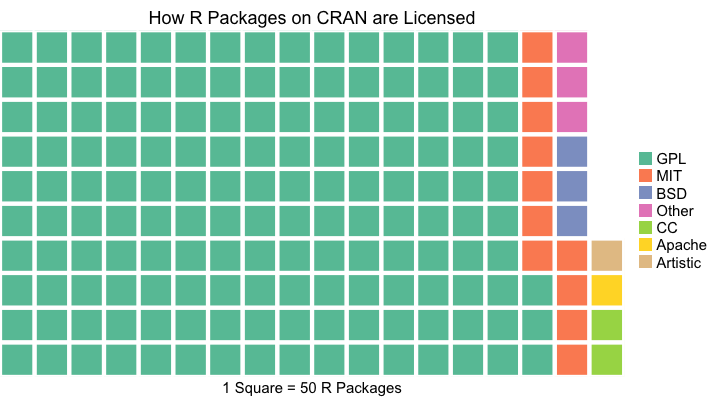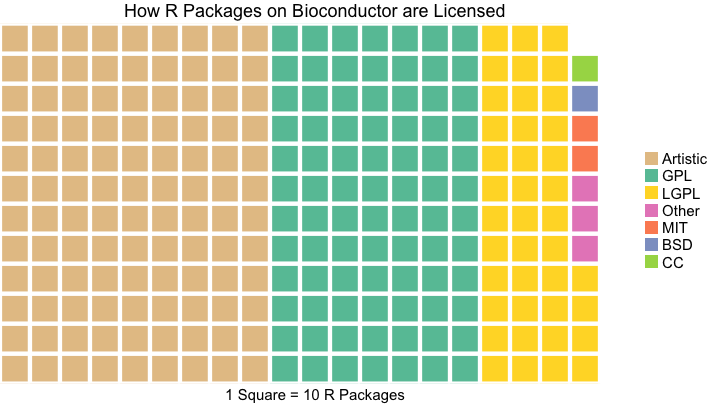# How R Packages are Licensed

I was researching R packages and I wanted to get a sense of how they are licensed on CRAN. I’ve also been wanting to take Bob Rudis’ waffle package for a spin since I read a study by Robert Kosara and Drew Skau saying that waffle charts (square shaped pie charts) are easier to read compared to pie charts. Below is a waffle chart illustrating the major open source licensing families among R packages on CRAN.

library(dplyr)
library(magrittr)
library(purrr)
library(waffle)
library(ggplot2)

# The sorting hat will map the License field in a DESCRIPTION file
# to an open source license family.
sorting_hat <- function(student, houses){
choice <- map_lgl(houses, grepl, x = student)
if(!any(choice)){
return("Other")
} else {
return((houses[choice]))
}
}

# Get the data from CRAN

map_chr(function(x){
sorting_hat(x, c("Apache", "Artistic", "CC", "BSD", "MIT", "GPL"))
})

# Tidy the data and plot!
summarize(Freq = sum(Freq)) %>%
ungroup() %>%
arrange(desc(Freq))

licenses <- license_tbl$Freq names(licenses) <- license_tbl$License

title = "How R Packages on CRAN are Licensed",
xlab = "1 Square = 50 R Packages") +
theme(legend.text=element_text(size=15), axis.title.x = element_text(size=15))## Update (Bioconductor)

Thanks for the idea Gabe. Below is a look at the licenses for packages on Bioconductor.

library(BiocInstaller)

names(bioc_table) %<>%
map_chr(function(x){
sorting_hat(x, c("Artistic", "CC", "BSD", "MIT", "LGPL", "GPL"))
})

# Tidy the data and plot!
bioc_tbl <- as.data.frame(bioc_table) %>%
summarize(Freq = sum(Freq)) %>%
ungroup() %>%
arrange(desc(Freq))

bioc <- bioc_tbl$Freq names(bioc) <- bioc_tbl$License

waffle(bioc/10, rows = 12,
title = "How R Packages on Bioconductor are Licensed",
xlab = "1 Square = 10 R Packages",
colors = c("#E5C494", "#66C2A5", "#FFD92F", "#E78AC3",
"#FC8D62", "#8DA0CB", "#A6D854")) +
theme(legend.text=element_text(size=15), axis.title.x = element_text(size=15))